# vs.eyeandcontacts.com

## Chapter 15 Introduction to Graphs Exercise 15.3

Question 1: Draw the graphs for the following tables of values, with suitable scales on the axes.
a) Cost of apples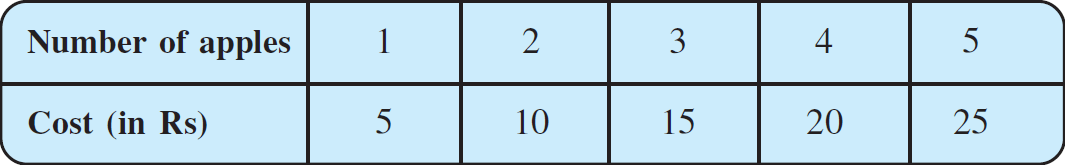b) Distance travelled by car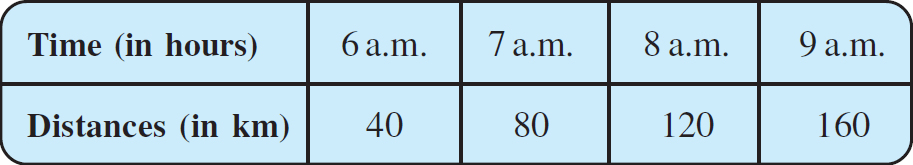i) How much distance did the car cover during the period 7.30 a.m. to 8 a.m.?
ii) What was the time when the car had covered a distance of 100 km since it’s start?
c) Interest on deposits for a year.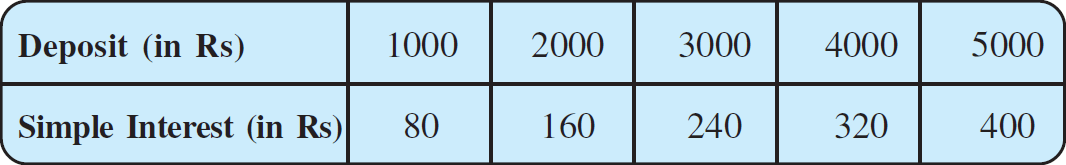i) Does the graph pass through the origin?
ii) Use the graph to find the interest on Rs 2500 for a year.
iii) To get an interest of Rs 280 per year, how much money should be deposited?

a)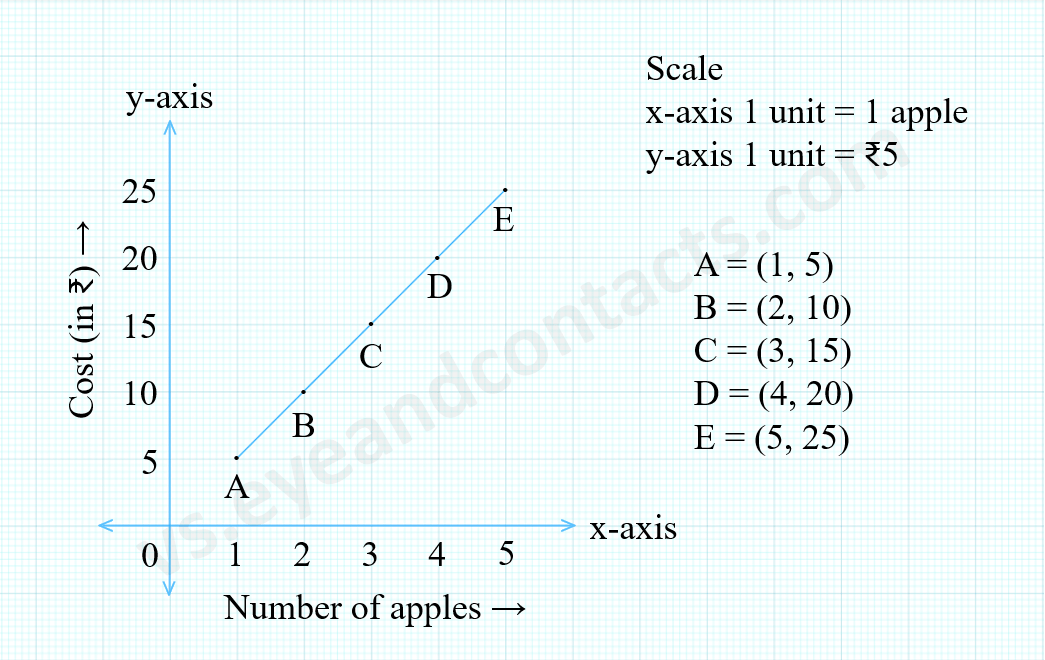b)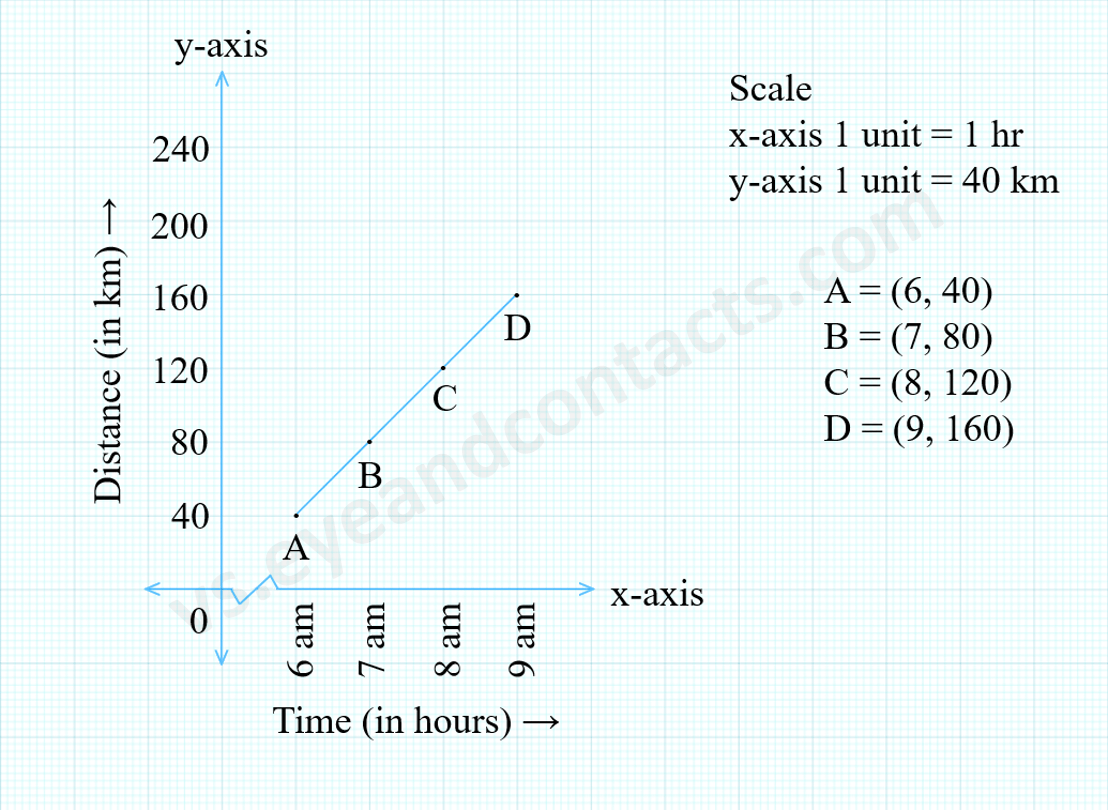i) The car covered 20 km during the period 7.30 a.m. to 8 a.m.
ii) The time when the car had covered a distance of 100 km since its start was 7:30 a.m.

c)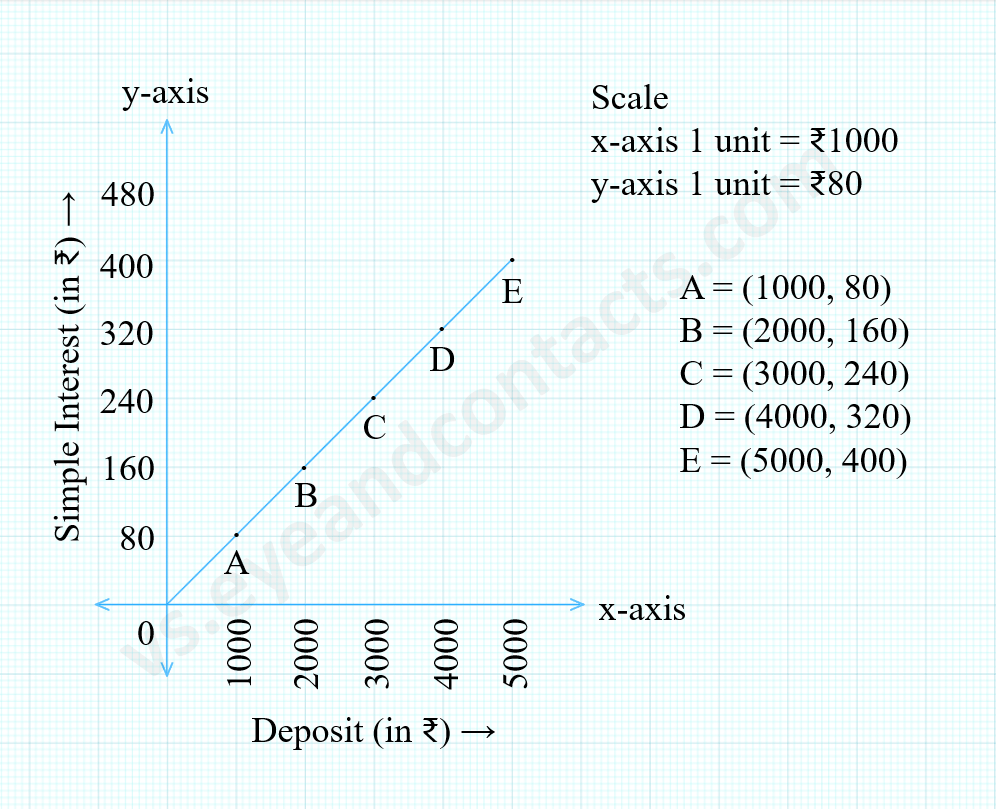i) Yes, the graph passes through the origin (0, 0).
ii) The interest received for deposit of ₹2500 a year is ₹200.
iii) To get an interest of ₹280 per year, ₹3500 should be deposited.

Question 2: Draw a graph for the following.
i)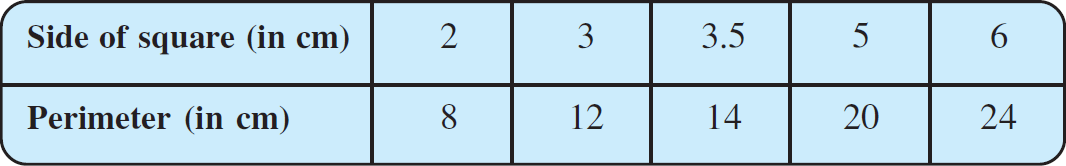Is it a linear graph?
ii)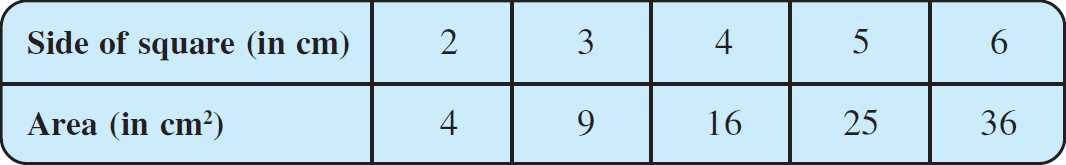Is it a linear graph?

i)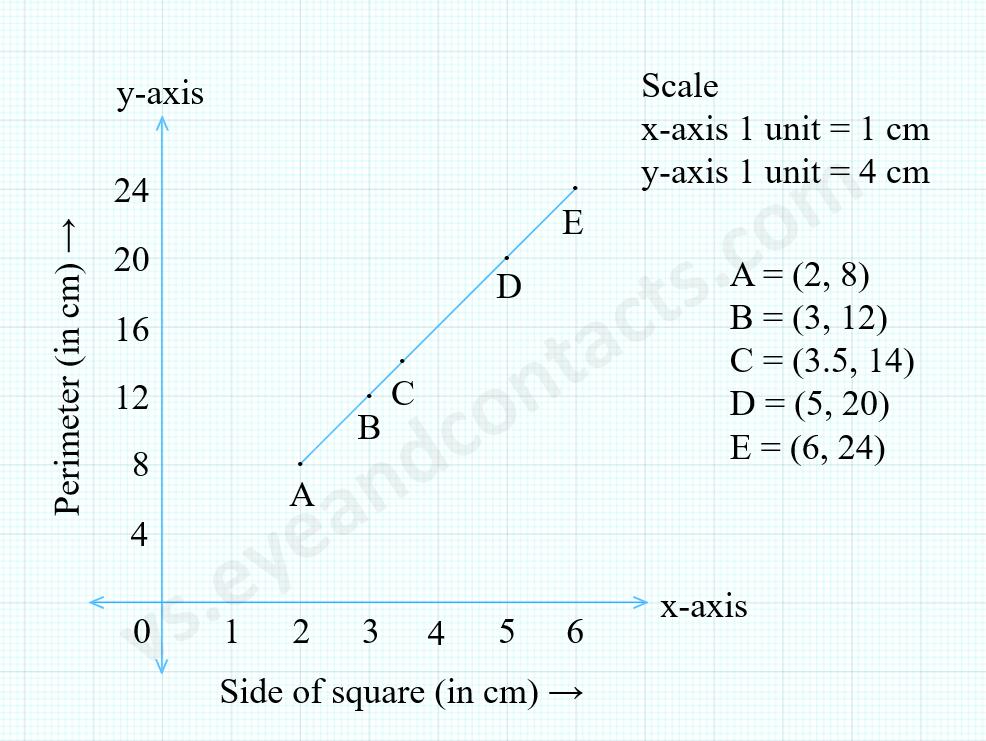Yes, it is a linear graph.

ii)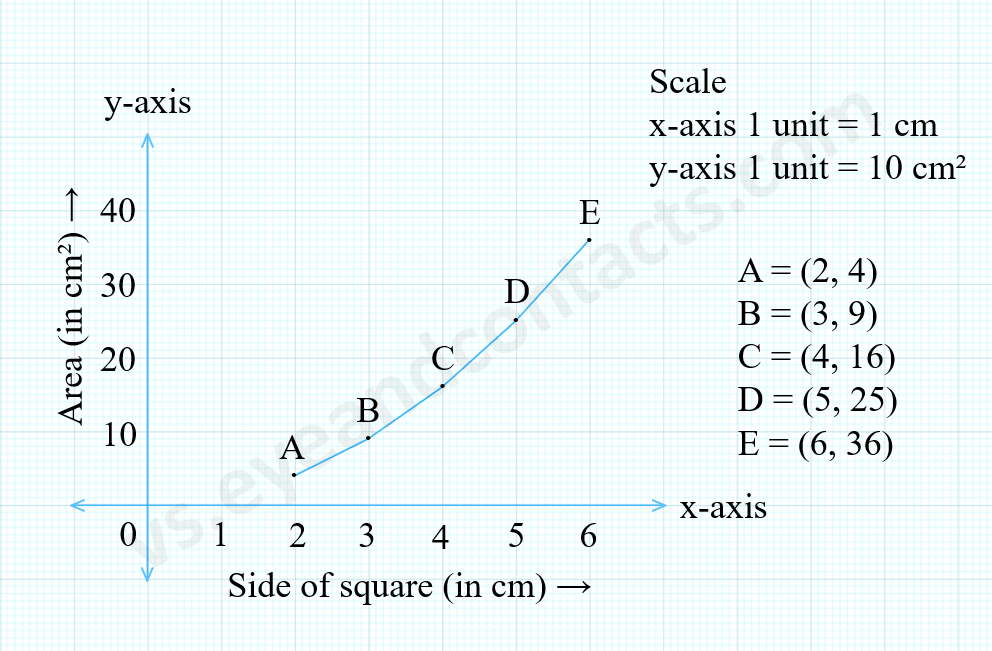No, it is not a linear graph because it is not a straight unbroken line.